Equilateral and Isoceles Triangle

Chapter 6 Class 7 Triangle and its Properties
Concept wise

We know that

Area of triangle =   1/2 × Base × Height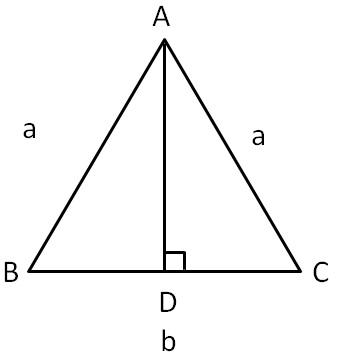Here,

Base = BC = b

### Finding height

Now,

In an isosceles triangle,

Median & Altitude are the same

So, D is mid-point of BC

∴ BD = DC = b/2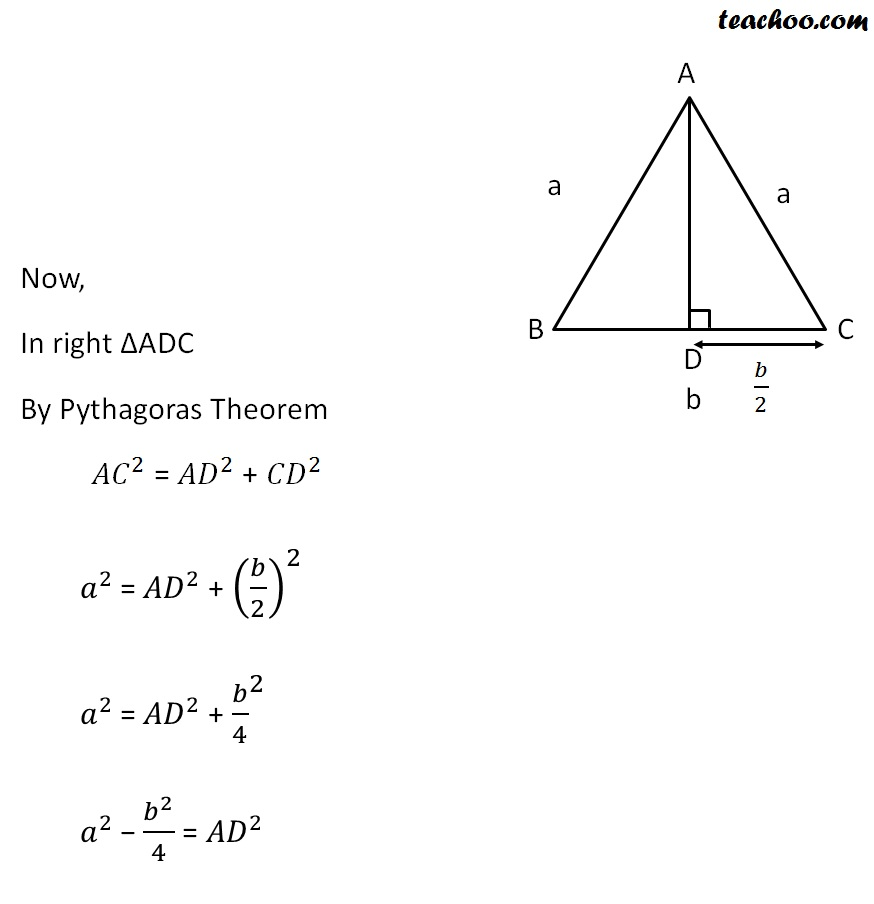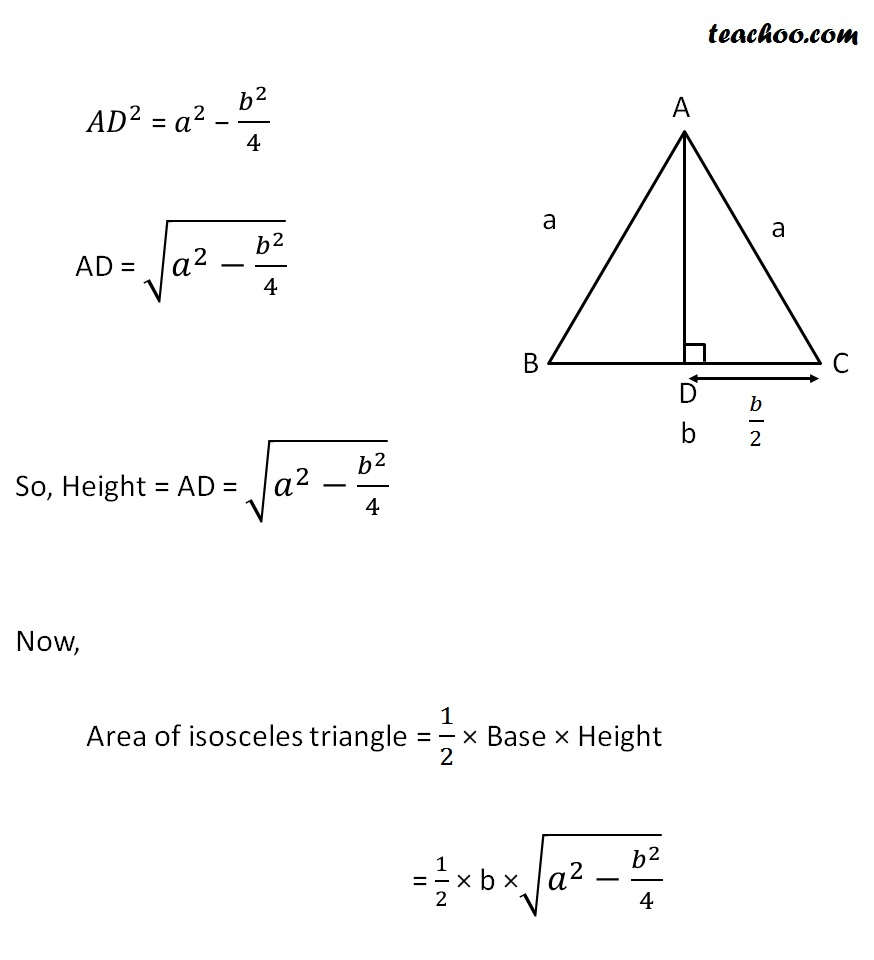### Find area of triangle ABC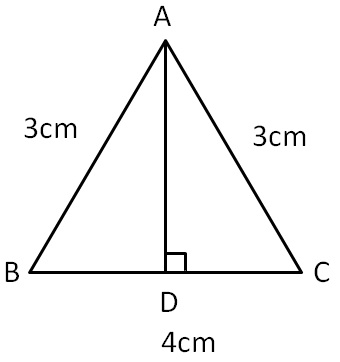We know that

Area of triangle =   1/2 × Base × Height

Here,

Base = BC = b = 4 cm

Height = h = AD = ?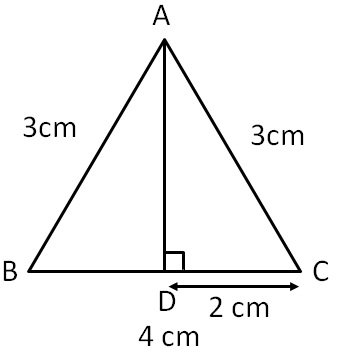Now,

In an isosceles triangle,

Median and altitude are the same

So, D is mid-point of BC

∴ BD = DC = 4/2

= 2cm

Now,

In ∆ADC, right angled at D

By Pythagoras theorem,

AC 2 = AD 2 + DC 2

(3) 2 = AD 2 + (2) 2

9 = AD 2 + 4

9 − 4 = AD 2So,

Height = h

= √5 cm

Now,

Area of a triangle = 1/2 × Base × Height

= 1/2 × 4 × √5

= 2 × √5

= 2√5 cm 2

### Find Area of triangle Δ ABC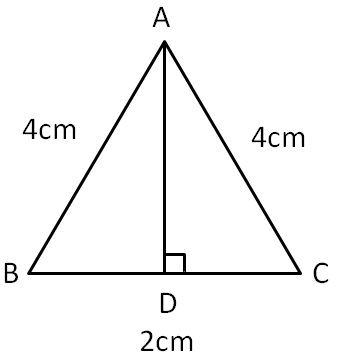We know that

Area of triangle =   1/2 × Base × Height

Here,

Base = b

= BC

= 2

Height = h

= ?

Finding height,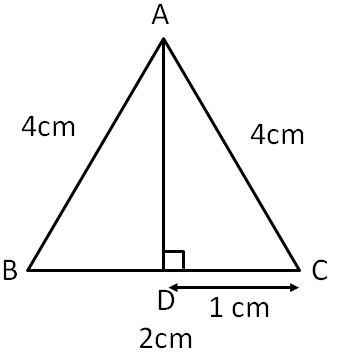Now,

In an isosceles triangle,

Median and altitude on base are the same.

So, D is the mid-point of BC

∴ BD = DC = 2/2

= 1 cmNow,

In ∆ADC, right angled at D

By Pythagoras theorem.

AC 2 = AD 2 + CD 2

(4) 2 = AD 2 + (1) 2

16 = AD 2 + 1

16 − 1 = AD 2

Thus,

Height = AD = √15 cm

Now,

Area of a triangle = 1/2 × Base × Height

= 1/2 × 2 × √15

= √15 cm 2

Learn in your speed, with individual attention - Teachoo Maths 1-on-1 Class